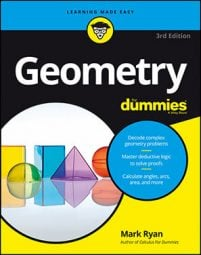##### Geometry For DummiesWhen you do an analytic proof, your first step is to draw a figure in the coordinate system and label its vertices. This figure will make the algebra part easier, when you have to prove something about the figure.

Here's an example. Say you're given the following proof: First, prove analytically that the midpoint of the hypotenuse of a right triangle is equidistant from the triangle's three vertices, and then show analytically that the median to this midpoint divides the triangle into two triangles of equal area.

Your first step in an analytic proof is to draw a figure in the x-y coordinate system and give its vertices coordinates. You want to put the figure in a convenient position that makes the math work out easily. For example, sometimes putting one of the vertices of your figure at the origin, (0, 0), makes the math easy because adding and subtracting with zeros is so simple. Quadrant I is also a good choice because all coordinates are positive there.

The figure you draw has to represent a general class of shapes, so you make the coordinates letters that can take on any values. You can't label the figure with numbers (except for using zero when you place a vertex at the origin or on the x- or y-axis) because that'd give the figure an exact size and shape—and then anything you proved would only apply to that particular shape rather than to an entire class of shapes.

Here's how you create your figure for the triangle proof in this example:

• Choose a convenient position and orientation for the figure in the x-y coordinate system. Because the x- and y-axes form a right angle at the origin (0, 0), that's the natural choice for the position of the right angle of the right triangle, with the legs of the triangle lying on the two axes. Then you have to decide which quadrant the triangle should go in. Unless you have some reason to pick a different quadrant, quadrant I is the best way to go.
• Choose suitable coordinates for the two vertices on the x- and y-axes. You'd often go with something like (a, 0) and (0, b) but here, because you're going to end up dividing these coordinates by 2 when you use the midpoint formula, the math will be easier if you use (2a, 0) and (0, 2b). Otherwise, you have to deal with fractions. Egad! The figure shows the final diagram.